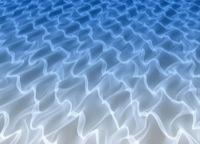Home

Astronomy research
Software instruments
Stellar equation of states
EOS with ionization
EOS for supernovae
Chemical potentials
Stellar atmospheres

Voigt Function
Jeans escape
Polytropic stars
Cold white dwarfs

Cold neutron stars
Stellar opacities
Neutrino energy loss rates
Ephemeris routines
Fermi-Dirac functions

Polyhedra volume
Plane - cube intersection
Coating an ellipsoid

Nuclear reaction networks
Nuclear statistical equilibrium
Laminar deflagrations
CJ detonations
ZND detonations

Fitting to conic sections
Unusual linear algebra
Derivatives on uneven grids

Supernova light curves
Exact Riemann solutions
1D PPM hydrodynamics
Hydrodynamic test cases
Galactic chemical evolution

Universal two-body problem
Circular and elliptical 3 body
The pendulum
Phyllotaxis

MESA
MESA-Web
FLASH

Zingale's software
Brown's dStar
GR1D code
Herwig's NuGRID
Meyer's NetNuc
Presentations
Illustrations
Public Outreach
Education materials
2023 ASU Solar Systems Astronomy
2023 ASU Energy in Everyday Life

AAS Journals
2023 AAS Peer Review Workshops
2023 MESA VI
2023 MESA Marketplace
2023 MESA Classroom
2023 Neutrino Emission from Stars
2023 White Dwarfs & 12C(α,γ)16O
2022 Earendel, A Highly Magnified Star
2022 Black Hole Mass Spectrum
2022 MESA in Don't Look Up
2021 Bill Paxton, Tinsley Prize

Contact: F.X.Timmes
my one page vitae,
full vitae,
research statement, and
teaching statement.

The tool contained in adiabatic_white_dwarf.tar.xz generates models of white dwarfs in hydrostatic equilibrium with an adiabatic temperature gradient for a general stellar equation of state. Such a model can be useful, for example, during the simmering phase of a white dwarf supernova progenitor. Starting from a given central density $\rho_c$, central temperature $T_c$, composition, and background temperature of the white dwarf $T_b$, this tool integrates the relevant equations of stellar structure \begin{equation} \begin{split} \frac{dr}{dm} & = \frac{3}{4 \pi r^2 \rho} \\ \frac{dP}{dm} & = \frac{dP}{dr} \frac{dr}{dm} = - \frac{G m \rho}{r^2} \frac{dr}{dm}\\ \frac{dT}{dm} & = \frac{dP}{dm} \ \frac{T}{P} \ \left ( \frac{\partial \ln T}{\partial \ln P} \right )_{\rm ad}\\ \frac{d\epsilon}{dm} & = q_{\rm eff} \ N_A \ \left ( \frac{1}{2} \ Y_{\rm 12}^2 \ \rho \ \lambda_{\rm 1212} \right ) \end{split} \label{eq1} \tag{1} \end{equation} where $r$ is the distance, $m$ is the mass, $P$ is the pressure, $N_A$ is the Avogadro number, $\lambda_{\rm 1212}$ is the C12+C12 reaction rate, and $q_{\rm eff}$ is the effective Q-value of the C12+C12 reaction (see Chamulak et al 2008 equation 6). Here are some examples with $T_b = 3 \times 10^8$ K. One can check the output files that the entropy is indeed constant in the adiabatic region ;)

Please cite the relevant references if you publish a piece of work that use these codes, pieces of these codes, or modified versions of them. Offer co-authorship as appropriate.